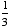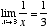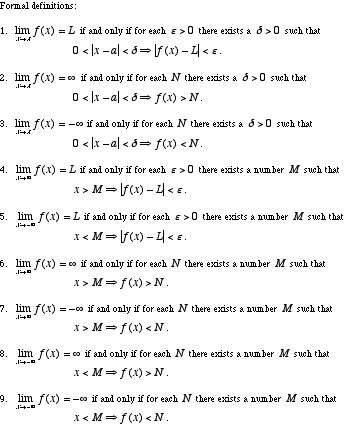index: click on a letter A B C D E F G H I J K L M N O P Q R S T U V W X Y Z A to Z index index: subject areas numbers & symbols sets, logic, proofs geometry algebra trigonometry advanced algebra & pre-calculus calculus advanced topics probability & statistics real world applications multimedia entrieswww.mathwords.com about mathwords website feedback

 Limit The value that a function or expression approaches as the domain variable(s) approach a specific value. Limits are written in the form. For example, the limit ofas x approaches 3 is. This is written.See also

 this page updated 19-jul-17 Mathwords: Terms and Formulas from Algebra I to Calculus written, illustrated, and webmastered by Bruce Simmons Copyright © 2000 by Bruce Simmons All rights reserved